Anúncio

# Geometric_algo.pdf

22 de Mar de 2023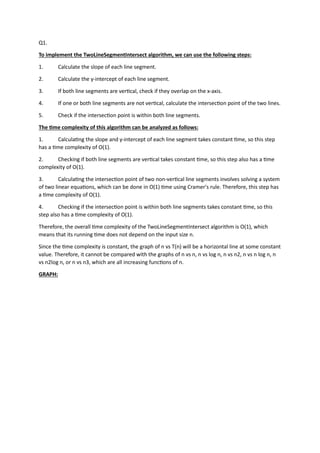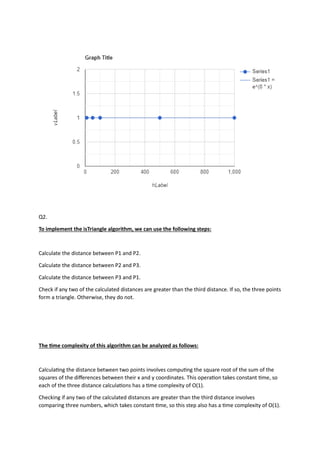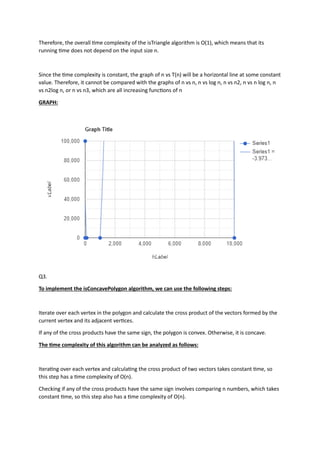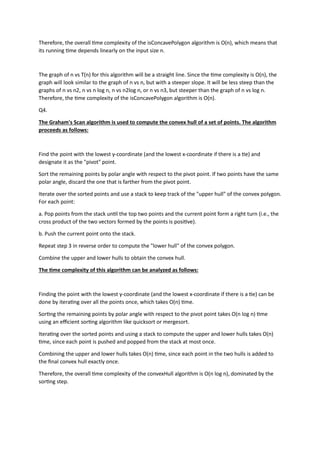Anúncio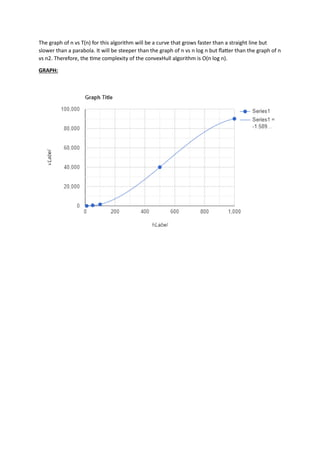Próximos SlideShares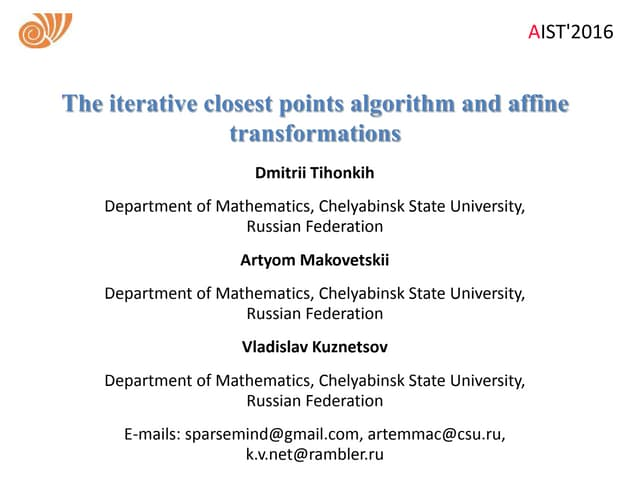Dmitrii Tihonkih - The Iterative Closest Points Algorithm and Affine Transfo...
Carregando em ... 3
1 de 5
Anúncio

### Geometric_algo.pdf

1. Q1. To implement the TwoLineSegmentIntersect algorithm, we can use the following steps: 1. Calculate the slope of each line segment. 2. Calculate the y-intercept of each line segment. 3. If both line segments are ver�cal, check if they overlap on the x-axis. 4. If one or both line segments are not ver�cal, calculate the intersec�on point of the two lines. 5. Check if the intersec�on point is within both line segments. The �me complexity of this algorithm can be analyzed as follows: 1. Calcula�ng the slope and y-intercept of each line segment takes constant �me, so this step has a �me complexity of O(1). 2. Checking if both line segments are ver�cal takes constant �me, so this step also has a �me complexity of O(1). 3. Calcula�ng the intersec�on point of two non-ver�cal line segments involves solving a system of two linear equa�ons, which can be done in O(1) �me using Cramer's rule. Therefore, this step has a �me complexity of O(1). 4. Checking if the intersec�on point is within both line segments takes constant �me, so this step also has a �me complexity of O(1). Therefore, the overall �me complexity of the TwoLineSegmentIntersect algorithm is O(1), which means that its running �me does not depend on the input size n. Since the �me complexity is constant, the graph of n vs T(n) will be a horizontal line at some constant value. Therefore, it cannot be compared with the graphs of n vs n, n vs log n, n vs n2, n vs n log n, n vs n2log n, or n vs n3, which are all increasing func�ons of n. GRAPH:
2. Q2. To implement the isTriangle algorithm, we can use the following steps: Calculate the distance between P1 and P2. Calculate the distance between P2 and P3. Calculate the distance between P3 and P1. Check if any two of the calculated distances are greater than the third distance. If so, the three points form a triangle. Otherwise, they do not. The �me complexity of this algorithm can be analyzed as follows: Calcula�ng the distance between two points involves compu�ng the square root of the sum of the squares of the diﬀerences between their x and y coordinates. This opera�on takes constant �me, so each of the three distance calcula�ons has a �me complexity of O(1). Checking if any two of the calculated distances are greater than the third distance involves comparing three numbers, which takes constant �me, so this step also has a �me complexity of O(1).
3. Therefore, the overall �me complexity of the isTriangle algorithm is O(1), which means that its running �me does not depend on the input size n. Since the �me complexity is constant, the graph of n vs T(n) will be a horizontal line at some constant value. Therefore, it cannot be compared with the graphs of n vs n, n vs log n, n vs n2, n vs n log n, n vs n2log n, or n vs n3, which are all increasing func�ons of n GRAPH: Q3. To implement the isConcavePolygon algorithm, we can use the following steps: Iterate over each vertex in the polygon and calculate the cross product of the vectors formed by the current vertex and its adjacent ver�ces. If any of the cross products have the same sign, the polygon is convex. Otherwise, it is concave. The �me complexity of this algorithm can be analyzed as follows: Itera�ng over each vertex and calcula�ng the cross product of two vectors takes constant �me, so this step has a �me complexity of O(n). Checking if any of the cross products have the same sign involves comparing n numbers, which takes constant �me, so this step also has a �me complexity of O(n).
4. Therefore, the overall �me complexity of the isConcavePolygon algorithm is O(n), which means that its running �me depends linearly on the input size n. The graph of n vs T(n) for this algorithm will be a straight line. Since the �me complexity is O(n), the graph will look similar to the graph of n vs n, but with a steeper slope. It will be less steep than the graphs of n vs n2, n vs n log n, n vs n2log n, or n vs n3, but steeper than the graph of n vs log n. Therefore, the �me complexity of the isConcavePolygon algorithm is O(n). Q4. The Graham's Scan algorithm is used to compute the convex hull of a set of points. The algorithm proceeds as follows: Find the point with the lowest y-coordinate (and the lowest x-coordinate if there is a �e) and designate it as the "pivot" point. Sort the remaining points by polar angle with respect to the pivot point. If two points have the same polar angle, discard the one that is farther from the pivot point. Iterate over the sorted points and use a stack to keep track of the "upper hull" of the convex polygon. For each point: a. Pop points from the stack un�l the top two points and the current point form a right turn (i.e., the cross product of the two vectors formed by the points is posi�ve). b. Push the current point onto the stack. Repeat step 3 in reverse order to compute the "lower hull" of the convex polygon. Combine the upper and lower hulls to obtain the convex hull. The �me complexity of this algorithm can be analyzed as follows: Finding the point with the lowest y-coordinate (and the lowest x-coordinate if there is a �e) can be done by itera�ng over all the points once, which takes O(n) �me. Sor�ng the remaining points by polar angle with respect to the pivot point takes O(n log n) �me using an eﬃcient sor�ng algorithm like quicksort or mergesort. Itera�ng over the sorted points and using a stack to compute the upper and lower hulls takes O(n) �me, since each point is pushed and popped from the stack at most once. Combining the upper and lower hulls takes O(n) �me, since each point in the two hulls is added to the ﬁnal convex hull exactly once. Therefore, the overall �me complexity of the convexHull algorithm is O(n log n), dominated by the sor�ng step.
5. The graph of n vs T(n) for this algorithm will be a curve that grows faster than a straight line but slower than a parabola. It will be steeper than the graph of n vs n log n but ﬂater than the graph of n vs n2. Therefore, the �me complexity of the convexHull algorithm is O(n log n). GRAPH:
Anúncio